Welcome to HiddenMysteries
Monday, September 23 2019 @ 04:32 AM CDT

# Last of the Ancient Wonders III : Pi, Phi and the Great Pyramid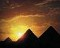Assem Deif investigates the values -- not the symbols -- of the last of the Wonders of the Ancient World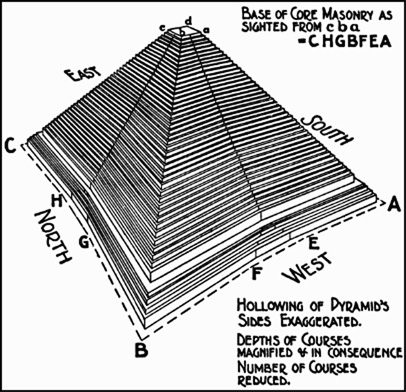We can forget all the ideas crediting Atlanteans or space aliens with building the Great Pyramid of Giza, and instead imagine ourselves travelling back in time in H G Wells's time machine to try and work out not how the ancient Egyptians built this enormous edifice, because this lies beyond our present understanding, but rather what we can best judge to be its most appropriate proportions. Then, however, there were no electronic calculators, only ropes and rods.

Constructing right angles at the four corners of a pyramid is easy. To do it, history tells us that the Egyptians were aware of the ratios 3:4:5 as the side-lengths of a right-angle triangle. Many old kingdom pyramids adhere to these ratios. The Egyptians also knew a rough value of Pi (the value, not the symbol) as the ratio between the circumference of any circle and its diameter. They worked out that 3 _ is less than Pi, and Pi is less than 3 1/7, i.e. Pi lies between the rational number 22/7 and the Babylonian value. This can be done by constructing a circle of diameter AB and laying the latter on its circumference, starting from A, once until C then D then E, to conclude that Pi is greater than 3. The remaining part EA from the circumference is laid down again on the diameter AB, so seven times EA is less than AB which in turn is less than eight times EA, or EA/AB is greater than 1/8 and less than 1/7.

To work out an Egyptian value for Pi from the dimensions of the Great Pyramid, they had a unit of length called the Royal Cubit (about 0.524m). By transforming the pyramid's height and base from western units (feet or metres) into cubits, it becomes evident that the designed height measures 280 cubits and the base 440. These figures have been worked out by Egyptologists, and in my view are the only plausible dimensions. Neither -- from the logical point of view -- were any fractions added to these values, considering the large dimensions of the structure. By dividing half the base by the height (cotangent of the slope angle) one reaches the ratio Pi/4. It then follows that the Egyptian value for Pi is 3.142857143 being equal exactly to 22/7, and this is the value, in our view, that was reported later by Archimedes, who studied in Alexandria.

What about the Egyptian value for the Golden Ratio Phi? In calculating the hypotenuse of the triangle of base 220c and height 280c, it is equal from the Pythagoras theorem to the square root of the sum of 48400 and 78400 (notice the resemblance between the digits) to be 356.0898763. Flinders Petrie noticed that the dimensions of this triangle were multiples of 4 cubits, since the base equals 55 times 4 whereas the hypotenuse is 89. It might be mere coincidence that the two numbers 55 and 89 are the 10th and 11th terms in the Fibonacci sequence, with Phi as the limit of its successive quotients. Thus the secant of the angle of inclination of the pyramid face on its base is 89/55 = 1.61818..., far more accurate than the one calculated in my last article. The Egyptian constants are now possibly: Pi = 22/7, Phi = 89/55.

Suggesting that it is a matter of coincidence that the Great Pyramid contains the above proportions could not be further from the truth. For one thing, Phi results automatically if one is to construct any right-angle triangle with dimensions a, b and c, satisfying c/b = b/a. It must have occurred to any mason, architect or engineer during the three millennia of Egyptian civilisation to seek the conditions under which the above proportionality criterion is satisfied. It follows from the Pythagoras theorem that the sides must satisfy a : b : c = 1 : root(Phi) : Phi.

Another pointer that the dimensions of the Great Pyramid are not the outcome of mere coincidence is the pyramid of Sneferu (Khufu's father) at Meidum, which keeps the same proportions between the dimensions of its inner triangle, namely (half-base : height : slant height = 137.5 : 175c : 222.5c). They are in the same proportions of Khufu's pyramid, but with a scale 1 : 1.6. It follows that Pi = 4 (137.5/175) = 22/7 and Phi = 222.5/137.5 = 89/55; leaving no room for coincidence. Again, the descending passage of the Red Pyramid at Dahshur has an angle of inclination of 26 like Khufu's, meaning that the Great Pyramid is only a continuation of the previous models.

The above value of Pi was later confirmed by F Gaendiger, who ingeniously deduced it from the well-known grid technique that the ancient Egyptians used as a background for their drawings. By imagining a grid of 10 x 10 squares, each measuring 1c x 1c, then by drawing a circle within the grid, the radius of the circle will measure 5c. The periphery of the circle will pass by 12 points of the grid; 4 points are given by the axes, while 8 further points are given by eight triangles measuring 3-4-5 cubits (arranged in the form of a Maltese cross). The 12 points divide the periphery of the circle into 4 short and 8 long arcs. By measuring these we find simple numbers: the long arc = 90 digits (28 digits make 1 cubit) and the small arc = 40 digits (or a few mms less). Hence the circumference of the circle measures 4x40 + 8x90 = 880 digits = 220/7 cubits. Dividing this measurement by the diameter 10 cubits gives exactly Pi = 22/7.

In the early 20th century archaeologists opened the tomb at Saqqara of the Egyptian architect Khesi-Ra, (a contemporary of Imhotep), who was the chief of doctors in the 27th century BC, the main writer and architect of the king and above all a carver. There were 11 boards in the tomb. At first Egyptologists accepted these panels as false doors, but later it was noticed that there was the proportion 1 : root(5) on the panel [Phi = (1+root(5))/2]. When the height of Khesi-Ra is divided into 18 even segments the navel is exactly where one would expect to find it according to the classic canon 11:18. The numbers 11 and 18 are approximately in the ratio of Phi; they belong to a sequence very much like the Fibonacci (Lucas) sequence composed of the numbers: 2, 1, 3, 4, 7, 11, 18, 29, etc., in which the ratio between two successive elements tends similarly to Phi.

After a comprehensive analysis by the method of proportions, Egyptologists had good cause to believe that Khesi-Ra's panels are the rules of harmony encoded by the language of geometry. The ingenious artist cut the panels with an accuracy that defies the precision of jewellers. These panels show the rule of the Golden Symphony in its broadest range of variations. However, Khesi-Ra is not the sole incident; when Vincent Brown measured the floor dimensions of the Birth Room in Luxor Temple he found it to be of Golden proportions. It does seem that whenever an ancient Egyptian artist sculpted or painted figures, his proportions were determined by a certain canon.

It is not easy, however, to persuade the Egyptology community that the ancient Egyptians knowingly used the Golden Ratio. The mere existence of the numbers in art and architecture is not necessarily proof that the artisan was aware of the ratio inherent in those numbers, but the vast number of incidents they match cannot be down to coincidence alone. Gay Robins wrote a book on the topic of proportions and the grid of 18 squares noting that it continued in from the Old Kingdom to the 26th Dynasty.

What if the Ancient Egyptians had wished to build a pyramid greater than the Great Pyramid while keeping the same proportions? What would be its dimensions? To assess this we can follow two different paths: first to assume that the dimensions of the new pyramid will be in the ratio 1.6:1 with respect to the Great Pyramid, being the same proportion between the latter and Meidum. In which case, the new dimensions become: Base length = 1.6x440c = 704c, Height = 1.6x280c = 448c. The other alternative is to follow Petrie's remark that the dimensions of the inner triangle of the Great Pyramid are multiples of 4 cubits. So, instead of considering the 9th and 10th terms of the Fibonacci series, we take the 10th and 11th terms -- as representatives in their ratio -- to the inverse of Phi, that is the terms 89 and 144, thus Base length= 89x4cx2 = 712c, Slant Height = 144x4c = 576c. It follows from the Pythagoras theorem that the pyramid height is equal to 452c. Both figures coincide with an error of only 1 percent. Both choices match because the number 1.6 is equal approximately to Phi.

To check the validity of this we should work in retrospect, i.e. try to calculate the dimensions of Meidum based on Petrie's remark. We must now consider the 8th and 9th terms of the sequence; being 34 and 55. Performing the same calculations for Meidum: Base length = 34x4cx2 = 272c, Slant Height = 55x4c = 220c of which the pyramid's height Pythagoras is 173c. Thus we have (half base: height: slant height = 136c : 173c : 220c). They compare well with the actual dimensions of Meidum. Again, the error amounts to no more than 1 percent. Petrie remarked that the dimensions of the Pi pyramids are multiple of 4 cubits may not be a coincidence, considering that the number 4 is sacred in ancient mythologies.

Strangely, Gantenbrink found that the heights of all construction points in the Great Pyramid were essentially either one 40th of the basic width of the pyramid or one 40th of their height. This is all the more surprising in that the base of 440 cubits when divided by 40 produces 11, and the height of 280 cubits when divided by 40 it produces 7. It might be a coincidence that the latter are the 5th and 6th terms in the Lucas sequence. This clear sub- division into 40th, Gantenbrink said, leads us to suspect that the architect of Khufu's Pyramid worked to a scale of 1:40 when placing his plan on papyrus. Still, the shifting of the lower construction point of the shafts from the pyramid axis amounts to exactly 22 cubits, i.e. 2 x 11 cubits. So it seems that the designers laid down a rectangular grid of 11 x 11 cubits in a ratio of 7 to 11 to be placed above the pyramid. The right, northern shaft is designed in a ratio of 11:7 grid points and the left, southern shaft in a square ratio of 7:7 grid points. If the reader projects the end points of the king's shafts on the pyramid base, he will end up with 3 additional rectangles of dimensions 7x11, 7x11 and 7x18. The lower part of the pyramid right down to the base continues to sub-divide in the ratio 7:11. The builders did not embark on a reckless building schema; the structure was already carefully planned before work commenced.

To proceed with our virtual pyramid, the one larger that Khufu's; the weight would equal 24 million tonnes, a stupendous weight contrasting with the still huge weight of about 6_ million tonnes for the Great Pyramid. To erect such a structure is not only difficult, but rather is out of the question; it would take some 75 years to build using the same workforce, a conclusion that is not even correct as the tasks achieved are not necessarily linearly proportional with the number of workers assigned -- administrating and assigning jobs would be all the more difficult. This 75-year period exceeds the reign of a king. In any case, the limestone blocks at the lower strata would probably not sustain such a weight.

It is apparent that the Egyptians were knowledgeable of some accurate values for both Pi and Phi (values, not symbols). Knowing Phi entails knowing the Pythagoras theorem; for the latter is the one which leads automatically to the ratios 1 : root (Phi) : Phi. Scholars believe the theorem was known hundreds of years before Pythagoras. It is even doubtful that the proof was credited to Pythagoras; the proof we know is the one given by Euclid I, 47, and is due to Euclid himself. Scientists have tried to elaborate on other proofs, among the simplest being to fill a square with 4 equal right-angle triangles each of dimensions (a:b:c) meeting at right angles such that the sum of their areas differs from that of the square by the area of a smaller square. As the area of the bigger square is c square, that of the 4 triangles is 4(1/2)ab and the one of the smaller square (a-b) square, it follows that c square = 2ab + (a-b) square from which one has that c square = a square + b square.

The Indian astronomer Bhaskara (c. 1150 AD) forwarded the simplest proof ever, one that could not have escaped the ancient Egyptians. He considered two squares, one rotating inside the other such that the vertices of the smaller one with side-length c touched the sides of the bigger one; 4 equal right-angle triangles are therefore constructed, each of dimension (a,b,c). Since the area of the bigger square is (a+b) square, that of the smaller square is c square and each of the 4 triangles has an area of (1/2) ab, it follows this time, that (a+b) square = 4(1/2) ab + c square; yielding the same result.

Of the 367 different proofs of Pythagoras theorem, one was forwarded by James Garfield. Five years before becoming president of the US, he discovered a fascinating proof, by equating the area of a trapezoid having 2 right angles with 3 inscribed right-angled triangles 2 equal small ones and one of having equal sides.

In the Berlin Papyrus (c. 1800 BC) we encounter the following problem: the area of a square of 100 is equal to that of two smaller squares. The side of one is 1/2 + 1/4 the side of the other. In other words, one seeks to solve equations of the sort: x square + y square = 100, which resembles very much the form of equations encountered in Pythagoras proofs.

One very unusual feature of the Great Pyramid is the concavity of its four faces, which makes the monument an 8-sided figure, rather than 4-sided like most of the pyramids. In other words, the four sides are hollowed to the inside. This concavity is executed to such an extraordinary degree of precision as to complement the mystery of the structure. From any ground position, it is invisible to the naked eye and only noticed from the air; indeed it was discovered by accident by a British air force pilot flying over the Giza Plateau in 1940 during the Spring Equinox (21 March). He did not miss had the opportunity and took a photograph. Petrie had already voiced a similar observation when he noticed a hollowing in the core masonry in the centre of each face such that the courses of the core had dips of as much as 1®. The concavity was presumably to prevent the casing stones from sliding, i.e. to better bond the nucleus to the casing. Another possibility was an aesthetic one: concave faces are more pleasing to the eye. The value of Phi, which is equal to the secant of the face angle of the inner triangle, is the same as the ratio between the slant height of the pyramid and half its base-length and this applies to every one of the 8 right-angle triangular faces. Each of the 8- sided faces confirms with the Golden Ratio proportion so that it is inscribed into a Golden Rectang le in which the height/base = Phi.

It is beyond any doubt that the dimensions of the Great Pyramid are not a coincidence conforming to Pi or Phi, but were chosen according to well-founded theories. It has become more and more obvious that the Giza Plateau in general and the Great Pyramid in particular were designed to encode sophisticated scientific knowledge. It is also likely that information abut the scientific skills of the ancient Egyptians has vanished in the whirlpool of time. Any script attesting to their mathematical or engineering capabilities must have perished owing to the fragile material on which they recorded their sciences.

Assem Deif is a professor of mathematics at Cairo University and Misr University for Science and Technology.

ahram.org.eg

http://egyptology.blogspot.com/2008/05/pi-phi-and-great-pyramid.html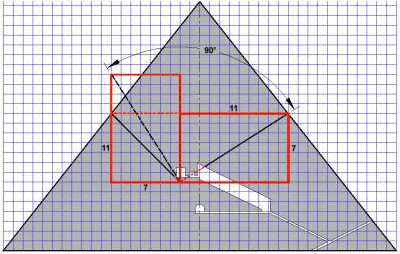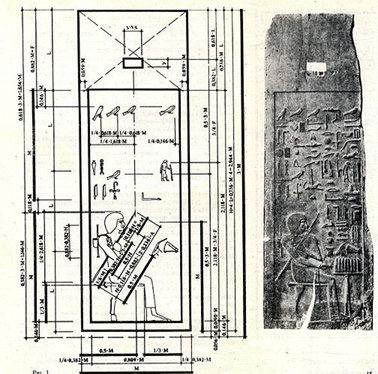A canon inside the pyramid (top left); the wooden panel of Khesi-Ra (above)

Check out these other Fine TGS sites

HiddenMysteries.com
HiddenMysteries.net
HiddenMysteries.org
TexasNationalPress.com
TGSPublishing.com
ReptilianAgenda.com
NationofTexas.com
Texas Nationalist Movement

* * * * * * * * * * * * * * * * * * * * * * * * * * * * * * * * * * * * * * *
A word from our sponsor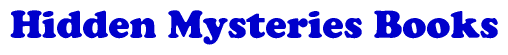## Think!No new stories